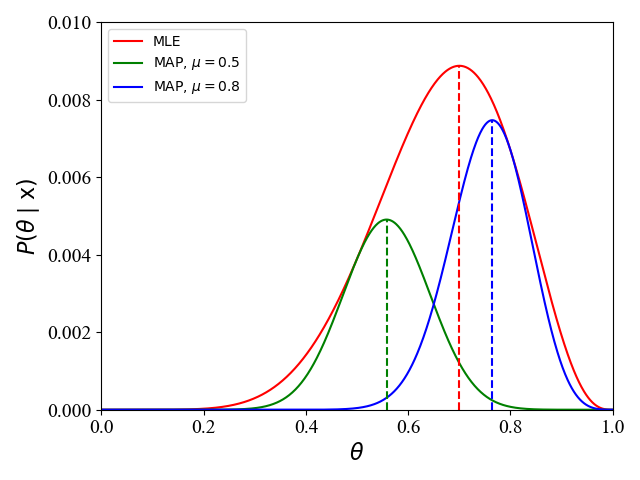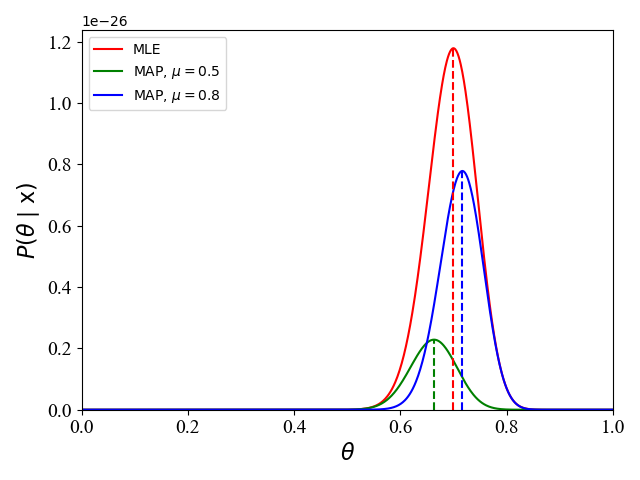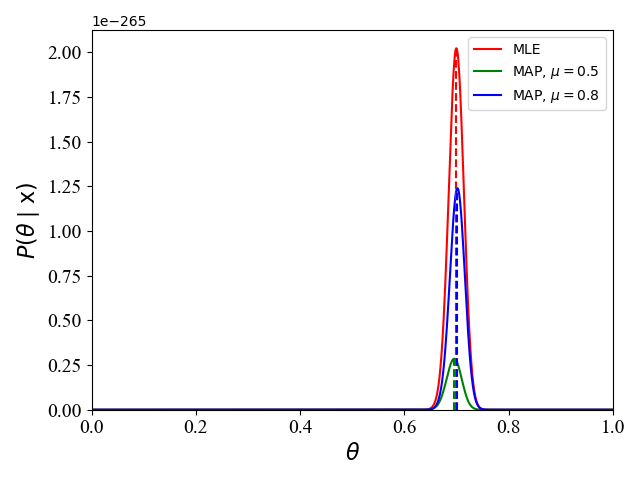• 基于EMS的最优线路参数估计模型，田君杨，韦化，当前电力系统实际情况，提出一种新的线路参数估计方法，考虑了测量函数、电压降落方程及测量数据约束方程，建立基于能量管理系统
• 对LLE降维算法中的邻域以及维度进行估计，得到最优参数，并附带参考文献可以学习，其中包含两份matlab代码可以使用
• 二乘是平方的意思，感觉最小二乘法就相当于均方误差（MSE）了，最小二乘法的思想是找到一组参数θ=(θ0,θ1,...,θn)\theta=(\theta_0, \theta_1, ..., \theta_n)θ=(θ0​,θ1​,...,θn​)使得∑i=1n(hθ(xi)−yi)...
参数估计(Parameter Estimate)就是通过一系列算法，来求出模型的最优参数。在各个机器学习深度学习的框架里，都变成了optimizer的活了。
其实这个名字很奇怪，但是在比较早的机器学习论文里都是这么叫的，我们重点来关注下里面涉及的一些算法。
这里主要关注的是

最小二乘法
梯度下降
牛顿法
拟牛顿法（未完成）

最小二乘法 Least Squares Method
二乘是平方的意思，感觉最小二乘法就相当于均方误差（MSE）了，最小二乘法的思想是找到一组参数$\theta=(\theta_0, \theta_1, ..., \theta_n)$使得$\sum_{i=1}^n(h_\theta(x_i)-y_i)^2$最小
具体求解时，通过代数法求解，假设模型为$h_\theta(x) = X\theta$，那么定义损失函数为$J(\theta) = \frac{1}{2}(X\theta-Y)^T(X\theta-Y)$，这里二分之一是为了计算方便。那么求解步骤如下：
$\frac{\partial J(\theta)}{\partial \theta} = X^T(X\theta-Y) = 0\\ \theta = (X^TX)^{-1}X^TY$
总结：

最小二乘法需要计算$(X^TX)^{-1}$，这个逆不一定存在；
当特征很多时，求逆的过程非常复杂；
当模型函数不是线性函数时，无法使用最小二乘法。

梯度下降 Gradient Descent
梯度下降是一种迭代求解的方法，主要思路是：
$\theta = \theta - \alpha \frac{\partial J(\theta)}{\partial \theta}$
其中，$\alpha$代表学习速率，也是迭代过程中的步长。
根据数据的不同，主要分为以下三种：

批量梯度下降，Batch Gradient Descent，直接在所有数据进行迭代计算
随机梯度下降，Stochastic Gradient Descent，每次选择在一条数据上进行迭代
小批量梯度下降，Mini-batch Gradient Descent，每次选择在一个小batch上进行迭代计算

总结：

优势：和最小二乘法相比，在没有解析解的时候也能进行迭代求解；
缺点：速度慢，没有最小二乘法快

牛顿法 Newton Method
牛顿法同样是使用近似求解，但是它的速度是比梯度下降法更快的。首先来看下牛顿法在求零点的时候的应用，对于函数$f(x)$求近似零点，假设当前的近似零点是$x_n$，要进一步求解下一个零点$x_{n+1}$，该怎么做呢？
首先，将在$x_n$处展开$f(x)$的二阶泰勒展开式得：
$f(x) = f(x_n) + f'(x_n)(x-x_n) + \frac{f''(x_n)}{2}(x-x_n)^2 \tag{1}$
此时，当前的零点满足
$f'(x_n) = 0 \tag{2}$
对(1)进行求导并结合(2)得
\begin{aligned} f'(x) & = 0 = f'(x_n) + f''(x_n)(x-x_n)\\ x & = x_n - \frac{f'(n)}{f''(n)} \end{aligned}
于是得到新的零点$x$，这里的$x$就是下一个零点$x_{n+1}$
现在把参数$x$扩展成向量，定义损失函数$f(x)$为
$\sum_{x \in \Bbb{R}^n}f(x)$
假设$f(x)$具有二阶连续偏导数，若第$k$次迭代值为$x^{(k)}$，则可将$f(x)$在$x^{(k)}$的附近进行二阶泰勒展开：
$f(x) = f(x^{(k)}) + g^T_k(x-x^{(k)}) + \frac{1}{2}(x-x^{(k)})^TH(x^{(k)})(x-x^{(k)})$
其中$g_k = g(x^{(k)})=\nabla f(x^{(k)})$是f(x)的梯度向量在点$x^{(k)}$的值，$H(x^{(k)})$是$f(x)$的海塞矩阵(Hessian Matrix)在点$x^{(k)}$的值，其中海塞矩阵的定义如下：
$H(x) = [\frac{\partial^2f}{\partial x_i \partial x_j}]_{n \times n}$
这里令$\nabla f(x)=0$，得到
\begin{aligned} g_k & + H_k(x-x^{(k)})= 0\\ x & = x^{(k)} - H_k^{-1}g_k \end{aligned}
这里将$H(x^{(k)})$简记为$H_k$，于是得到了牛顿法。
总结：

优势：速度快
缺点：海塞矩阵的逆矩阵计算太耗时了

改进方法：拟牛顿法


展开全文• 在分析非线性扩散性质的基础上，结合图像的结构特征和视觉特性，提出了一种最优梯度阈值和最佳扩散时间尺度的估计方法。实验结果表明该方法与最小均方误差准则、信噪比准则和相关系数最小准则相比，具有更好的稳定性...
• EM算法在进行GMM参数估计时怎么避免陷入局部最优，用禁忌搜索算法可以吗？有会的吗？求大佬帮主如题，有会的帮帮我吧，有偿的，Q875884675em算法 禁忌搜索算法 算法
• 全书共分七章，包括时间序列ARMA模型和状态空间模型，最小二乘法参数估计，ARMA时间序列预报，经典Kalman滤波理论及多传染器最优信息融合Kalman滤波理论，基于现代时间序列分析方法的最优滤理论及最优信息融合滤波...
• 7.3 最优检测器设计及假性检验 二值假设检验 检测问题 随机检测器 检测概率矩阵 检测器设计的多准则表述 标量化 检测问题： 假设X是随机变量，在中取值，其概率密度分布和参数的取值有关，对的m个可能值，X的...
7.3 最优检测器设计及假性检验

二值假设检验

检测问题
随机检测器
检测概率矩阵
检测器设计的多准则表述
标量化
检测问题：

假设X是随机变量，在中取值，其概率密度分布和参数的取值有关，对的m个可能值，X的概率密度分布可以由矩阵表征，其元素为：，即矩阵的第j列对于参数值的概率分布。

对的m个可能值称为m个假设，我们需要从假设中猜想哪个是正确的，这个问题称为假设检验，而假设检验也可以看成观测到X的某个取值，然后判断不寻常事件（某种假设为一种常规事件，而其他假设均对应不寻常事件）是否发生，如果发生了，是哪一个不寻常事件。于是假设检验问题又称为检测问题。

二值假设检验，即一种特殊的检验问题，即m=2，只有两个假设。这里X是随机变量，且，假设1：X由概率密度分布产生；假设2：X由概率密度分布产生；

随机检测器

随机检测器可以定义为一个矩阵，其元素为，其中是的猜想值，k是X的观测值。T可以理解为，如果我们得到观测值X=k，那么检测器以概率给出估计值。显然T满足两个约束，。

在二值假设检验中：如果观测到X=k，估计假设1的概率为，估计假设2的概率为。

如果矩阵T的元素要么是0，要么是1，则矩阵T是确定性检测器。

检测概率矩阵

定义检测矩阵D=TP，于是有，即是当时，猜想为的概率。

二值假设检验中：

其中表示X是由分布p产生但却被估计为假设2（由分布q产生）的概率，表示X是由分布q产生但却被估计为假设1（由分p产生）的概率。

检测器设计的多准则表述

对于二值假设检验问题，检测器设计的目标就是极小化和，即

而对于一般的假设检验，检测器设计的目标是极小化，即

标量化

对于二值假设检验问题的标量化，即加上一个惩罚权重，即：

其中表示列向量Tp的第2行的元素。

解析解：

对于一般的假设检验问题的标量化，即引入权系数将目标函数加权求和，即

其中

检验问题等价于：

目标函数还可以描述为一系列的线性函数的和：

其中是的第k列。

于是可以通过分别求解下面的线性规划来求解检验问题。

极小极大检测器

对于二值假设检验，极小极大检测器，即求解：

来源：https://blog.csdn.net/wangchy29/article/details/87465799

展开全文• 7.3最优检测器设计及假性检验 二值假设检验 检测问题 随机检测器 检测概率矩阵 检测器设计的多准则表述 标量化 检测问题： 假设X是随机变量，在中取值，其概率密度分布和参数的取值有关，对的m个可能值，X的...
7.3最优检测器设计及假性检验

二值假设检验

检测问题
随机检测器
检测概率矩阵
检测器设计的多准则表述
标量化
检测问题：

假设X是随机变量，在中取值，其概率密度分布和参数的取值有关，对的m个可能值，X的概率密度分布可以由矩阵表征，其元素为：，即矩阵的第j列对于参数值的概率分布。

对的m个可能值称为m个假设，我们需要从假设中猜想哪个是正确的，这个问题称为假设检验，而假设检验也可以看成观测到X的某个取值，然后判断不寻常事件（某种假设为一种常规事件，而其他假设均对应不寻常事件）是否发生，如果发生了，是哪一个不寻常事件。于是假设检验问题又称为检测问题。

二值假设检验，即一种特殊的检验问题，即m=2，只有两个假设。这里X是随机变量，且，假设1：X由概率密度分布产生；假设2：X由概率密度分布产生；

随机检测器

随机检测器可以定义为一个矩阵，其元素为，其中是的猜想值，k是X的观测值。T可以理解为，如果我们得到观测值X=k，那么检测器以概率给出估计值。显然T满足两个约束，。

在二值假设检验中：如果观测到X=k，估计假设1的概率为，估计假设2的概率为。

如果矩阵T的元素要么是0，要么是1，则矩阵T是确定性检测器。

检测概率矩阵

定义检测矩阵D=TP，于是有，即是当时，猜想为的概率。

二值假设检验中：

其中表示X是由分布p产生但却被估计为假设2（由分布q产生）的概率，表示X是由分布q产生但却被估计为假设1（由分p产生）的概率。

检测器设计的多准则表述

对于二值假设检验问题，检测器设计的目标就是极小化和，即

而对于一般的假设检验，检测器设计的目标是极小化，即

标量化

对于二值假设检验问题的标量化，即加上一个惩罚权重，即：

其中表示列向量Tp的第2行的元素。

解析解：

对于一般的假设检验问题的标量化，即引入权系数将目标函数加权求和，即

其中

检验问题等价于：

目标函数还可以描述为一系列的线性函数的和：

其中是的第k列。

于是可以通过分别求解下面的线性规划来求解检验问题。

极小极大检测器

对于二值假设检验，极小极大检测器，即求解：


展开全文• 分析线性最小二乘与非线性最小二乘在三轴加速计参数估计上的效果。 包含报告和matlab程序。
• 本文以线性时不变或系统参数预先确定的单传感器系统为对象, 研究带有不确定随机无序量测约束下的最优网络化估计问题. 基于线性时不变或参数预先确定系统的滤波器系数矩阵离线计算和线性最小方差估计的线性加权求和...
• 然后,拟合出检测器最优控制参数的经验公式,经验公式符合数值结果;最后,将α-AMF与改进的α-AMF的恒虚警率特性和检测性能进行对比分析.研究结果表明,在复合高斯环境下,基于SCM-NSCM估计的α-AMF受杂波尖峰的影响小于...
• 该方法首先利用Chan算法计算定位初始位置，在初始位置处泰勒级数展开得到位置估计量的线性模型，并求取误差加权矩阵、系数矩阵及协方差矩阵等参数；然后采用加权最小二乘法对最终位置进行最优无偏估计，同时推导出...到达时间差 定位误差
• 准则获得最优尺度参数, 并选取具有高阶消失矩的小波基函数进行小波变换, 从而可以获得数字调 制信号符号率信息. 仿真结果表明, 新算法在数据量较少和低信噪比条件下, 估计性能优于经典 Haar 小波方法, 具有较好的普...
• 例如，我们用二项分布去拟合多次投掷硬币的情况，计算该二项分布的最优参数（出现正面的概率 θ\thetaθ）就是参数估计。 下面，我们介绍在机器学习中常用的参数估计：极大似然估计 (Maximum Likelihood Estimation,...
参数估计：给定一个数据集，我们希望用一个给定的分布去拟合该数据集的分布，确定该分布的参数的过程就是参数估计。例如，我们用二项分布去拟合多次投掷硬币的情况，计算该二项分布的最优参数（出现正面的概率 $\theta$）就是参数估计。
下面，我们介绍在机器学习中常用的参数估计：极大似然估计 (Maximum Likelihood Estimation, MLE)，最大后验概率估计 (Maximum A Posteriori, MAP)。在此之前，我们介绍一下参数估计中常用的一些概念.

频率学派 VS. 贝叶斯学派

频率学派：事件本身是服从某种参数$\theta$固定的分布。频率学派认为概率即是频率，某次得到的样本$\mathrm x$只是无数次可能的试验结果的一个具体实现，样本中未出现的结果不是不可能出现，只是这次抽样没有出现而已。在参数估计中，频率学派的代表是最大似然估计 MLE。
贝叶斯学派：参数$\theta$也是随机分布的。贝叶斯学派认为只能依靠得到的样本$\mathrm x$去做推断，而不能考虑那些有可能出现而未出现的结果。同时，贝叶斯学派引入了主观的概念，认为一个事件在发生之前，人们应该对它是有所认知，即先验概率$p(\theta)$，然后根据样本$\mathrm x$ 通过贝叶斯定理来得到后验概率$p(\theta\mid\mathrm x)$。在参数估计中，贝叶斯学派的代表是最大后验概率估计 MAP。

概率 VS. 统计
概率与统计可以看成是互逆的概念。在http://stanford.edu/~lanhuong/refresher/notes/probstat-section3.pdf中对概念与统计推断作了简要概述：

• The basic problem of probability is: Given the distribution of the data, what are the properties (e.g. its expectation) of the outcomes (i.e. the data)?
• The basic problem of statistical inference is the inverse of probability: Given the outcomes, what can we say about the process that generated the data?

对于在机器学习中的常见问题，这里的data就是我们的训练样例，且机器学习的目的就是 say about the process that generated the data, 即学习生成训练样例的模型。

似然函数 VS. 概率函数
似然函数和概率函数的数学表达式一样，只是以不同的角度看待该函数表达式：

若$\theta$已知，$\mathrm x$是变量，$P(\mathrm x\mid\theta)$ 被称为概率函数；
若$\mathrm x$已知，$\theta$是变量，$P(\mathrm x\mid\theta)$ 被称为似然函数；

​        一般为了保持符号的一致性，似然函数也经常写作$L(\theta\mid\mathrm x)$。

极大似然估计 (MLE)
最大似然估计MLE的思想是，寻找使得观测到的数据出现概率最大的参数$\theta$。
对于抛硬币来说，在一次抛硬币时，其结果的概率分布如下：
\begin{aligned} P(\mathrm x_i\mid\theta)&=\begin{cases} \theta,\quad\quad\hspace{4mm}\mathrm x_i=1\\ 1-\theta,\quad\mathrm x_i=0 \end{cases}\\ &=\theta^{\mathrm x_i}(1-\theta)^{1-\mathrm x_i} \end{aligned}\tag{1}
其中$\mathrm x_i=1$表示第$i$抛硬币时正面朝上。那么抛$N$次硬币，其结果为$\{\mathrm x_1,\mathrm x_2,\cdots,\mathrm x_N\}$的概率为
$P(\mathrm x_1,\mathrm x_2,\cdots,\mathrm x_N\mid\theta)=\prod\limits_{i=1}^{N}\theta^{\mathrm x_i}(1-\theta)^{1-\mathrm x_i}\tag{2}$
MLE就是寻找最优的$\theta$最大化公式(2)的概率，即求解
$\theta^\star=\arg\max_{\theta}\prod\limits_{i=1}^{N}\theta^{\mathrm x_i}(1-\theta)^{1-\mathrm x_i}\tag{3}$
对于优化问题(3)，我们一般考虑将其转为对数目标函数，一方面可以将连乘转化为加和，防止计算溢出；另一方面使得目标函数更加精炼，便于通过求导求解最优解(连乘的导数不易计算)。为此，优化问题(3)可以转化为：
$\theta^\star=\arg\max_{\theta}\sum\limits_{i=1}^{N}\log{\theta^{\mathrm x_i}(1-\theta)^{1-\mathrm x_i}}=\arg\max_{\theta}\sum\limits_{i=1}^{N}\mathrm x_i\log{\theta}+(1-\mathrm x_i)\log{(1-\theta)}\tag{4}$
对(4)的目标函数对$\theta$求导，并令导数为0 (目标函数为凹函数，在导数为0点取得极值)，我们有：
$\sum\limits_{i=1}^{N}\mathrm x_i\frac{1}{\theta}+(1-\mathrm x_i)\frac{-1}{1-\theta}=0\rightarrow\theta=\frac{\sum\nolimits_{i=1}^{N}\mathrm x_i}{\sum\nolimits_{i=1}^{N}1}\tag{5}$
公式(5)的结果比较符合直观：比如抛硬币10次，发现5次正面朝上，我们就说出现正面朝上的概率为0.5. 但是，也可能出现7次正面朝上的情况，这时我们说出现正面朝上的概率为0.7，显然这时与实际情况不符合(假定硬币是均匀的)。也就是说，当试验次数较少时，使用最大似然函数时的误差会较大。

上式(1)-(5)详细推导了离散的二项分布的最大似然估计(5)。对于常用的连续分布正态分布$\mathcal N(\mu,\sigma^2)$，我们只需要将公式(2)中的连乘项改为正态分布的概率密度函数，然后通过对数、求导为零，可以得到其最大似然估计为：
$\mu=\frac{1}{N}\sum\limits_{i=1}^{N}x_i\tag{6}$
$\sigma^2=\frac{1}{N}\sum\limits_{i=1}^{N}(x_i-\mu)^2\tag{7}$
其中，我们这里假设总共有$N$个样本，$x_1,x_2,\cdots,x_N$。

最大后验概率估计 (MAP)
在最大后验概率MAP中，参数$\theta$被看作为一个随机变量，服从某种概率分布，被称为先验概率$P(\theta)$。
还是以上述抛硬币为例，考虑到先验概率，优化问题(3)被改写为：
$\theta^\star=\arg\max_{\theta}\prod\limits_{i=1}^{N}\theta^{\mathrm x_i}(1-\theta)^{1-\mathrm x_i}p(\theta)\tag{8}$
同样地，将公式(8)进行对数化可得：
\begin{aligned} \theta^\star&=\arg\max_{\theta}\sum\limits_{i=1}^{N}\log{\theta^{\mathrm x_i}(1-\theta)^{1-\mathrm x_i}}p(\theta)\\&=\arg\max_{\theta}\sum\limits_{i=1}^{N}\mathrm x_i\log{\theta}+(1-\mathrm x_i)\log{(1-\theta)}+\log p(\theta)\tag{9} \end{aligned}
一般地，我们假设硬币是均匀地，即$p(\theta=\frac{1}{2})=1$，即此时参数$\theta$时一个固定的未知量。此时，对(8)的目标函数对$\theta$求导，并令导数为0，我们可以得到和公式(5)一样的结果。这说明，当先验分布为均匀分布时，MLE等价于MAP。但是，在最大后验概率中，我们可以假设$\theta$是服从某一概率分布的。这里我们假设$\theta\sim N(\mu,\sigma)$，即
$p(\theta)=\frac{1}{\sqrt{2\pi}\sigma}e^{-\frac{(\theta-\mu)^2}{2\sigma^2}}\tag{10}$
将公式(10)带入公式(9)可得：
$\theta^\star=\arg\max_{\theta}\sum\limits_{i=1}^{N}\mathrm x_i\log{\theta}+(1-\mathrm x_i)\log{(1-\theta)}+\log{\frac{1}{\sqrt{2\pi}\sigma}}-\frac{(\theta-\mu)^2}{2\sigma^2}\tag{11}$
注意：由于正态分布的概率密度函数(10)是关于$\theta$ 的凹函数，公式(4)也是凹函数，所以公式(11)中的目标函数也是凹函数，所以我们可以利用导数为0取得最优的参数值$\theta^\star$。但是此时，我们一般无法得到如公式(5)一样简洁的解析表达式。在下面的具体实例中，我们直接给出目标函数的图像，从而可以形象地直接确定其最优解。对于比较复杂的目标函数，我们就需要借助其他迭代算法来求解了。
对于一个具体实例 ($\mu=0.5,\sigma=0.1$，事件$\mathrm x$为10次试验有7次为正面朝上)，此时问题(8)中的目标函数为：
$P(\theta\mid\mathrm x)=P(\mathrm x\mid\theta)P(\theta)=\theta^7(1-\theta)^3\frac{10}{\sqrt{2\pi}}e^{-50(\theta-0.5)^2}\tag{10}$
我们可以画出其函数曲线如下：图1

从图1中可以看出，当我们采用不同的先验概率分布时 ($\mu=0.5,\mu=0.8$)，最终得到的参数也不同 ($\theta^\star=0.56,\theta^\star=0.76$)。在这里，我们假设硬币是均匀的，即正面朝上的概率为$\theta=0.5$，此时与MLE相比 ($\theta=0.7$)，MAP的性能时好时坏，也就是说，MAP的性能与先验概率分布的选取有关。

等效情况
如前面所提及的，当先验概率为均匀分布时，MLE和MAP等效，因为此时$\theta$服从均匀分布，没有提供有效的关于$\theta$的先验信息。MLE和MAP等效的另一种情况就是：在频率学派所代表的MLE，当观测数据变大时(例子中对应抛硬币次数)，这时观测数据的本身就提供了足够的信息，先验概率的影响变得微不足道，此时MLE和MAP等效，即最终估计的参数值$\theta^\star$相同。如下图2和3，表示了当100次抛硬币70次为正面，和1000次抛硬币700次为正面时，对应的似然函数和后验概率函数：图2图3

附录
下面给出图1-3的python源代码，由于代码简单，所以就没有注释
图1：
# -*- encoding: utf-8 -*-
"""
@File    : Parameter_Estimation_fig001.py
@Time    : 2020/5/31 14:46
@Author  : tengweitw
@Email   : tengweitw@foxmail.com
"""

import numpy as np
import matplotlib.pyplot as plt

# Set the format of labels
def LabelFormat(plt):
ax = plt.gca()
plt.tick_params(labelsize=14)
labels = ax.get_xticklabels() + ax.get_yticklabels()
[label.set_fontname('Times New Roman') for label in labels]
font = {'family': 'Times New Roman',
'weight': 'normal',
'size': 16,
}
return font

sigma=0.1
mu1=0.5
mu2=0.8

theta=np.linspace(0,1,1000)
p_theta_x1=theta**7*(1-theta)**3/(np.sqrt(2*np.pi)*sigma)*np.exp(-np.square(theta-mu1)/2/np.square(sigma))
p_theta_x2=theta**7*(1-theta)**3/(np.sqrt(2*np.pi)*sigma)*np.exp(-np.square(theta-mu2)/2/np.square(sigma))

p_theta_x0=theta**7*(1-theta)**3/(np.sqrt(2*np.pi)*sigma)

p_max_ind0=np.argmax(p_theta_x0)
print(theta[p_max_ind0])

p_max_ind1=np.argmax(p_theta_x1)
print(theta[p_max_ind1])

p_max_ind2=np.argmax(p_theta_x2)
print(theta[p_max_ind2])

plt.figure()
plt.plot(theta,p_theta_x0,'r-')
plt.plot(theta,p_theta_x1,'g-')
plt.plot(theta,p_theta_x2,'b-')

plt.plot([theta[p_max_ind0],theta[p_max_ind0]],[0,p_theta_x0[p_max_ind0]],'r--')
plt.plot([theta[p_max_ind1],theta[p_max_ind1]],[0,p_theta_x1[p_max_ind1]],'g--')
plt.plot([theta[p_max_ind2],theta[p_max_ind2]],[0,p_theta_x2[p_max_ind2]],'b--')

plt.legend(["MLE",r"MAP, $\mu=0.5$",r"MAP, $\mu=0.8$"])

font = LabelFormat(plt)
plt.xlabel(r'$\theta$', font)
plt.ylabel(r'$P(\theta\mid\mathrm{x})$', font)
plt.xlim(0,1)
plt.ylim(0,0.01)
plt.show()

图2-3的源代码：
# -*- encoding: utf-8 -*-
"""
@File    : Parameter_Estimation_fig002.py
@Time    : 2020/5/31 16:01
@Author  : tengweitw
@Email   : tengweitw@foxmail.com
"""

import numpy as np
import matplotlib.pyplot as plt

# Set the format of labels
def LabelFormat(plt):
ax = plt.gca()
plt.tick_params(labelsize=14)
labels = ax.get_xticklabels() + ax.get_yticklabels()
[label.set_fontname('Times New Roman') for label in labels]
font = {'family': 'Times New Roman',
'weight': 'normal',
'size': 16,
}
return font

sigma=0.1
mu1=0.5
mu2=0.8

theta=np.linspace(0,1,1000)
# Here to change 700 300 to 70 30 vice verse
p_theta_x1=theta**70*(1-theta)**30/(np.sqrt(2*np.pi)*sigma)*np.exp(-np.square(theta-mu1)/2/np.square(sigma))
p_theta_x2=theta**70*(1-theta)**30/(np.sqrt(2*np.pi)*sigma)*np.exp(-np.square(theta-mu2)/2/np.square(sigma))
p_theta_x0=theta**70*(1-theta)**30/(np.sqrt(2*np.pi)*sigma)

p_max_ind0=np.argmax(p_theta_x0)
print(theta[p_max_ind0])

p_max_ind1=np.argmax(p_theta_x1)
print(theta[p_max_ind1])

p_max_ind2=np.argmax(p_theta_x2)
print(theta[p_max_ind2])

plt.figure()
plt.plot(theta,p_theta_x0,'r-')
plt.plot(theta,p_theta_x1,'g-')
plt.plot(theta,p_theta_x2,'b-')

plt.plot([theta[p_max_ind0],theta[p_max_ind0]],[0,p_theta_x0[p_max_ind0]],'r--')
plt.plot([theta[p_max_ind1],theta[p_max_ind1]],[0,p_theta_x1[p_max_ind1]],'g--')
plt.plot([theta[p_max_ind2],theta[p_max_ind2]],[0,p_theta_x2[p_max_ind2]],'b--')

plt.legend(["MLE",r"MAP, $\mu=0.5$",r"MAP, $\mu=0.8$"])

font = LabelFormat(plt)
plt.xlabel(r'$\theta$', font)
plt.ylabel(r'$P(\theta\mid\mathrm{x})$', font)
plt.xlim(0,1)
plt.ylim(ymin=0)
plt.show()



展开全文• 论文研究-重尾分布二阶参数的半参数估计.pdf, 重尾分布二阶参数在极值理论中扮演着重要的角色，尤其是重尾指数估计中门限的最优选取，以及重尾指数降偏差估计的渐近偏差...
• 参数估计的计算方法极大后验(MAP)及拉普拉斯逼近基于马尔可夫链的蒙特卡洛参数推断(MCMC)期望极大化(EM) （参数估计所有内容） 极大后验(MAP)及拉普拉斯逼近 极大后验估计： MAP是通过确定后验分布的极大值得到的，...
• 基于最优邻域的混沌时间序列预测法，吕王勇，王会琦，在混沌时间序列的局域预测法中，邻域的选择与计算没有充分应用已有的信息，提出结合非参数估计最优窗宽的思想，对任意形式的拟
• 导论 信号处理的基本任务是利用观测... 参数化估计与系统模型的辨识密切相关，其主要理论是优化理论，即被估计的参数应在某种准侧下是最优的，以及如何获得最优参数估计。 非参数化方法不假定数据服从某种特定...
• 最大似然估计与贝叶斯参数估计 前言 上一篇讲到了贝叶斯决策理论，其与贝叶斯估计是两种不同的思想。前者是根据先验概率P(ωi)P(ωi)P(\omega_i)和类条件概率密度p(x|ωi)p(x|ωi)p(x|\omega_i)来设计最优分类器...
• 该方法利用DCT变换中图像块低频能量的差异将DCT系数划分成平滑和纹理两类,并分别对这两类数据进行参数估计以实现基于最大似然比的最优检测.实验表明该方法较原有的整体参数估计和基于频段分类的参数估计在性能上有...
• 本文以已实现核估计作为波动率非参数估计的代表，构建了一种能自动从实际数据中确定最优窗宽的算法.理论分析的结果表明：算法具有稳定性，其所确定的窗宽是最优窗宽的无偏一致估计量，收敛速度为O（n-1/5）.实际数据...
• 第一章 介绍 第二章 阵列和空域滤波器 第三章 线性阵列和孔径的合成 第四章 平面阵列和孔径 第五章 空时过程的特性 第六章 最优波形估计 第七章 自适应波束形成...第八章 参数估计1 第九章 参数估计2 主要参考文献 后记
• 二是网络参数估计，即已知网络结构，估计每个条件概率分布的参数。 不含隐变量的参数估计 如果图模型中不包含隐变量，即所有变量都是可观测的，那么网络参数一般可以直接通过最大似然来进行估计。 含隐变量的参数...
• 针对永磁同步电机（PMSM）的关键参数估计，提出了一种基于学习策略的动态粒子群优化算法（DPSO-LS），其中参数估计模型中考虑了电压源逆变器（VSI）的非线性。可以与其他机器参数同时估算在DPSO-LS算法中，设计了一...Dynamic
• 在有限的样本下，如何判定哪个估计最优，概率论中有两种常用的principle：MLE（Maximum likelihood estimation），MAP(Maximum a posteriori estimation)。由于估计的是一个确定的参数值，MLE和MAP称为点估计。事实...
• 在现实的通信系统中,许多自然的以及人为的噪声是非高斯脉冲的。针对噪声的非高斯性引起的高斯假设下设计的最优接收机性能的显著退化。...并进行了模型仿真和模型参数估计。结果表明， α稳定分布更能描述信道中的噪音
• 我们是利用先验概率 P(c)P(c)P(c) 和 类条件概率密度 p(x∣c)p(x|c)p(x∣c) 来设计最优分类器，但是在实际应用中，通常是拿不到概率结构的全部知识的，因此我们就需要利用这些训练样本来估计问题中涉及的先验概率和...
• 基于傅里叶分析（DFT）的谱参数估计方法已被广泛用于测量多普勒天气雷达降水速度，已经证明对于较小的归一化谱宽[(σN)]而言该方法几乎最优。然而该方法局限于地基和空基多普勒天气雷达中较小的[σN][(σN)]。研究...
• 请教各位，我用R对别人论文的损失率数据，用beta 分布拟合，参数估计结果是3.97和3.85，而原文用STATA估计的结果是6.36和5.7。做K-S检验，我的P值是0.01835，而原文的P值是0.3657，说明似乎原文的估计结果更为合理。......